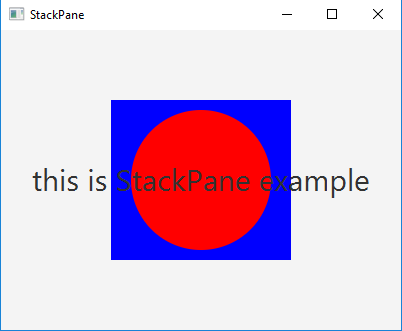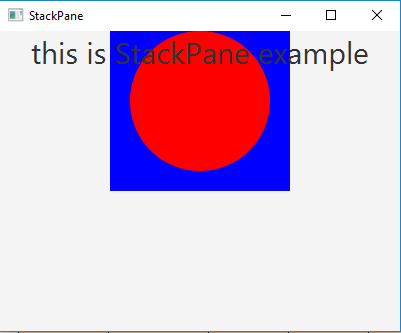# JavaFX | StackPane Class

StackPane class is a part of JavaFX. StackPane class lays out its children in form of a stack. The new node is placed on the top of the previous node in a StackPane. StackPane class inherits Pane Class.

Constructors of the class:

1. StackPane(): Creates a new empty StackPane.
2. StackPane(Node… c): Creates a new StackPne with specified nodes.

Commonly Used Methods:

Method Explanation
getAlignment() Returns the alignment of the StackPane.
getAlignment(Node c) Returns the node’s alignment.
getMargin(Node c) Returns the insets of the node.
setAlignment(Node n, Pos v) Sets the alignment of the node which is a part of StackPane.
setAlignment(Pos v) Sets the alignment of the StackPane.
setMargin(Node n, Insets v) Sets the margin of the node which is a part of StackPane.

Below programs illustrate the use of StackPane Class:

1. Java Program to create a StackPane, add circle, label, rectangle and add it to the stage: In this program we are creating a Label named label, a Rectangle named rectangle and a Circle named circle. Then set the font of the StackPane using the setFont() function. Now set the fill of the rectangle and circle using the setFill() function. We will then create a StackPane named stack_pane and add rectangle, circle and label. Create a scene and add the stack_pane to the scene. Add this scene to the stage and call the show() function to display the final results.

 `// Java Program to create a StackPane, ` `// add circle, label, rectangle ` `// and add it to the stage ` `import` `javafx.application.Application; ` `import` `javafx.scene.Scene; ` `import` `javafx.scene.control.*; ` `import` `javafx.scene.layout.*; ` `import` `javafx.stage.Stage; ` `import` `javafx.event.ActionEvent; ` `import` `javafx.scene.paint.*; ` `import` `javafx.scene.canvas.*; ` `import` `javafx.scene.text.*; ` `import` `javafx.scene.Group; ` `import` `javafx.scene.shape.*; ` ` `  `public` `class` `StackPane_1 ``extends` `Application { ` ` `  `    ``// launch the application ` `    ``public` `void` `start(Stage stage) ` `    ``{ ` ` `  `        ``try` `{ ` ` `  `            ``// set title for the stage ` `            ``stage.setTitle(``"StackPane"``); ` ` `  `            ``// create a label ` `            ``Label label = ``new` `Label(``"this is StackPane example"``); ` ` `  `            ``// set Font for label ` `            ``label.setFont(``new` `Font(``30``)); ` ` `  `            ``// create a circle ` `            ``Circle circle = ``new` `Circle(``100``, ``100``, ``70``); ` ` `  `            ``// set fill for  the circle ` `            ``circle.setFill(Color.RED); ` ` `  `            ``// create Rectangle ` `            ``Rectangle rectangle = ``new` `Rectangle(``100``, ``100``, ``180``, ``160``); ` ` `  `            ``// set fill for rectangle ` `            ``rectangle.setFill(Color.BLUE); ` ` `  `            ``// create a stack pane ` `            ``StackPane stack_pane = ``new` `StackPane(rectangle, circle, label); ` ` `  `            ``// create a scene ` `            ``Scene scene = ``new` `Scene(stack_pane, ``400``, ``300``); ` ` `  `            ``// set the scene ` `            ``stage.setScene(scene); ` ` `  `            ``stage.show(); ` `        ``} ` ` `  `        ``catch` `(Exception e) { ` ` `  `            ``System.out.println(e.getMessage()); ` `        ``} ` `    ``} ` ` `  `    ``// Main Method ` `    ``public` `static` `void` `main(String args[]) ` `    ``{ ` ` `  `        ``// launch the application ` `        ``launch(args); ` `    ``} ` `} `

Output:2. Java Program to create a StackPane, add the circle, label, rectangle and then set the alignment of the StackPane and add it to the stage: In this program we are creating a Label named label, a Rectangle named rectangle and a Circle named circle. Then set the font of the StackPane using the setFont() function. Set fill of the rectangle and circle using the setFill() function. Now create a StackPane named stack_pane and add rectangle, circle, and label. Set the alignment of the stack_pane using setAlignment() function. Create a scene and add the stack_pane to the scene. Finally add this scene to the stage and call the show() function to display the results.

 `// Java Program to create a StackPane,  ` `// add the circle, label, rectangle and ` `// then set the alignment of the StackPane ` `// and add it to the stage ` `import` `javafx.application.Application; ` `import` `javafx.scene.Scene; ` `import` `javafx.scene.control.*; ` `import` `javafx.scene.layout.*; ` `import` `javafx.stage.Stage; ` `import` `javafx.geometry.*; ` `import` `javafx.scene.paint.*; ` `import` `javafx.scene.canvas.*; ` `import` `javafx.scene.text.*; ` `import` `javafx.scene.Group; ` `import` `javafx.scene.shape.*; ` ` `  `public` `class` `StackPane_2 ``extends` `Application { ` ` `  `    ``// launch the application ` `    ``public` `void` `start(Stage stage) ` `    ``{ ` ` `  `        ``try` `{ ` ` `  `            ``// set title for the stage ` `            ``stage.setTitle(``"StackPane"``); ` ` `  `            ``// create a label ` `            ``Label label = ``new` `Label(``"this is StackPane example"``); ` ` `  `            ``// set Font for label ` `            ``label.setFont(``new` `Font(``30``)); ` ` `  `            ``// create a circle ` `            ``Circle circle = ``new` `Circle(``100``, ``100``, ``70``); ` ` `  `            ``// set fill for  the circle ` `            ``circle.setFill(Color.RED); ` ` `  `            ``// create Rectangle ` `            ``Rectangle rectangle = ``new` `Rectangle(``100``, ``100``, ``180``, ``160``); ` ` `  `            ``// set fill for rectangle ` `            ``rectangle.setFill(Color.BLUE); ` ` `  `            ``// create a stack pane ` `            ``StackPane stack_pane = ``new` `StackPane(rectangle, circle, label); ` ` `  `            ``// set alignement for the stack pane ` `            ``stack_pane.setAlignment(Pos.TOP_CENTER); ` ` `  `            ``// create a scene ` `            ``Scene scene = ``new` `Scene(stack_pane, ``400``, ``300``); ` ` `  `            ``// set the scene ` `            ``stage.setScene(scene); ` ` `  `            ``stage.show(); ` `        ``} ` ` `  `        ``catch` `(Exception e) { ` ` `  `            ``System.out.println(e.getMessage()); ` `        ``} ` `    ``} ` ` `  `    ``// Main Method ` `    ``public` `static` `void` `main(String args[]) ` `    ``{ ` ` `  `        ``// launch the application ` `        ``launch(args); ` `    ``} ` `} `

Output:3. Note: The above programs might not run in an online IDE please use an offline compiler.

Attention reader! Don’t stop learning now. Get hold of all the important Java and Collections concepts with the Fundamentals of Java and Java Collections Course at a student-friendly price and become industry ready.

My Personal Notes arrow_drop_upCheck out this Author's contributed articles.

If you like GeeksforGeeks and would like to contribute, you can also write an article using contribute.geeksforgeeks.org or mail your article to contribute@geeksforgeeks.org. See your article appearing on the GeeksforGeeks main page and help other Geeks.

Please Improve this article if you find anything incorrect by clicking on the "Improve Article" button below.

Article Tags :
Practice Tags :

Be the First to upvote.

Please write to us at contribute@geeksforgeeks.org to report any issue with the above content.